Most Affordable JEE | NEET | 8,9,10 Preparation by Kota's Top IITian Doctor Faculties

# NCERT Solutions for Class 11 Biology chapter 17 Breathing and Exchange of Gases PDF - eSaral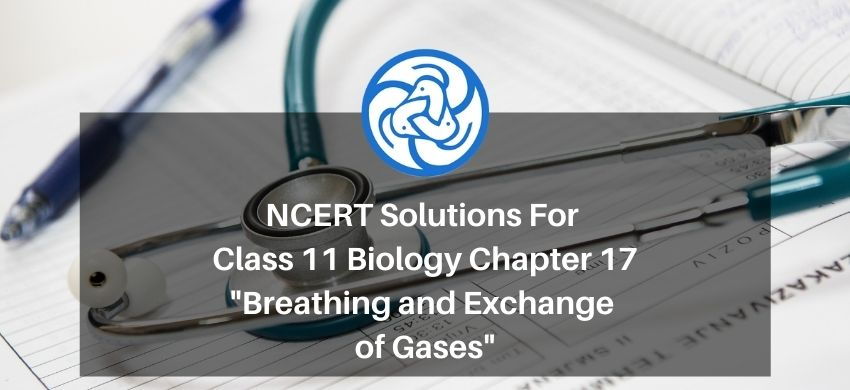# NCERT Solutions for Class 11 Biology chapter 17 Breathing and Exchange of Gases PDF

Hey, are you a class 11 student and looking for ways to download NCERT Solutions for Class 11 Biology chapter 17 Breathing and Exchange of Gases PDF? If yes. Then read this post till the end.

In this article, we have listed NCERT Solutions for Class 11 Biology chapter 17 Breathing and Exchange of Gases in PDF that are prepared by Kota’s top Doctor’s Faculties by keeping Simplicity in mind.

If you want to learn and understand class 11 Biology chapter 17 "Breathing and Exchange of Gases" in an easy way then you can use these solutions PDF.

NCERT Solutions helps students to Practice important concepts of subjects easily. Class 11 Biology solutions provide detailed explanations of all the NCERT questions that students can use to clear their doubts instantly.

If you want to score high in your class 11 Biology Exam then it is very important for you to have a good knowledge of all the important topics, so to learn and practice those topics you can use eSaral NCERT Solutions.

In this article, we have listed NCERT Solutions for Class 11 Biology chapter 17 Breathing and Exchange of Gases PDF that you can download to start your preparations anytime.

So, without wasting more time Let’s start.

### Download NCERT Solutions for Class 11 Biology chapter 17 Breathing and Exchange of Gases PDF

Question 1: Define vital capacity. What is its significance?

Solution. Vital capacity is the maximum volume of air that can be exhaled after a maximum inspiration. It is about $3.5-4.5$ litres in the human body. It promotes the act of supplying fresh air and getting rid of foul air, thereby increasing the gaseous exchange between the tissues and the environment.

Question 2: State the volume of air remaining in the lungs after a normal breathing.

Solution. jolume of air remaining in the lungs after a normal expiration is known as functional residual capacity (FRC). It includes expiratory reserve volume (ERV) and residual volur (RV). ERV is the maximum volume of air that can be exhaled after a normal expiration. It is about $1000 \mathrm{~mL}$ to $1500 \mathrm{~mL} . \mathrm{RV}$ is the volume of air remaining in the lungs after maximum expiration. It is about $1100 \mathrm{~mL}$ to $1500 \mathrm{~mL}$.

$\therefore \mathrm{FRC}=\mathrm{ERV}+\mathrm{RV}$

$\cong 1500+1500$

$\cong 3000 \mathrm{~mL}$

Functional residual capacity of the human lungs is about $2500-3000 \mathrm{~mL}$.

Question 3: Diffusion of gases occurs in the alveolar region only and not in the other parts of respiratory system. Why?

Solution. Each alveolus is made up of highly-permeable and thin layers of squamous epithelial cells. Similarly, the blood capillaries have layers of squamous epithelial cells. Oxygen-rich ail enters the body through the nose and reaches the alveoli. The deoxygenated (carbon dioxide-rich) blood from the body is brought to the heart by the veins. The heart pumps it to the lungs for oxygenation. The exchange of $\mathrm{O}_{2}$ and $\mathrm{CO}_{2}$ takes place between the blood capillaries surrounding the alveoli and the gases present in the alveoli.

Thus, the alveoli are the sites for gaseous exchange. The exchange of gases takes place by simple diffusion because of pressure or concentration differences. The barrier between the alveoli and the capillaries is thin and the diffusion of gases takes place from higher partial pressure to lower partial pressure. The venous blood that reaches the alveoli has lower partial pressure of $\mathrm{O}_{2}$ and higher partial pressure of $\mathrm{CO}_{2}$ as compared to alveolar air. Hence, oxygen diffuses into blood. Simultaneously, carbon dioxide diffuses out of blood and into the alveoli.

Question 4 : What are the major transport mechanisms for $\mathrm{CO}_{2}$ ? Explain.

Solution. Plasma and red blood cells transport carbon dioxide. This is because they are readily soluble in water.

(1) Through plasma:

About $7 \%$ of $\mathrm{CO}_{2}$ is carried in a dissolved state through plasma. Carbon dioxide combines with water and forms carbonic acid.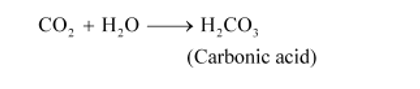Since the process of forming carbonic acid is slow, only a small amount of carbon dioxide is carried this way.

(2) Through RBCs:

About $20-25 \%$ of $\mathrm{CO}_{2}$ is transported by the red blood cells as carbaminohaemoglobin. Carbon dioxide binds to the amino groups on the polypeptide chains of haemoglobin and forms a compound known as carbaminohaemoglobin

(3) Through sodium bicarbonate:

About $70 \%$ of carbon dioxide is transported as sodium bicarbonate. As $\mathrm{CO}_{2}$ diffuses into the blood plasma, a large part of it combines with water to form carbonic acid in the presence of the enzyme carbonic anhydrase. Carbonic anhydrase is a zinc enzyme that speeds up the formation of carbonic acid. This carbonic acid dissociates into bicarbonate $\left(\mathrm{HCO}_{3}^{-}\right)$and hydrogen ions $\left(\mathrm{H}^{+}\right)$.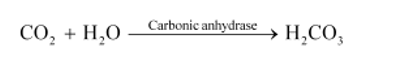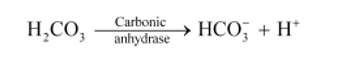Question 5: What will be the $\mathrm{pO}_{2}$ and $\mathrm{pCO}_{2}$ in the atmospheric air compared to those in the alveolar air?

(i) $\mathrm{pO}_{2}$ lesser, $\mathrm{pCO}_{2}$ higher

(ii) $\mathrm{pO}_{2}$ higher, $\mathrm{pCO}_{2}$ lesser

(iii) $\mathrm{pO}_{2}$ higher, $\mathrm{pCO}_{2}$ higher

(iv) $\mathrm{pO}_{2}$ lesser, $\mathrm{pCO}_{2}$ lesser

Solution. Answer: (ii) $\mathrm{pO}_{2}$ higher, $\mathrm{pCO}_{2}$ lesser

The partial pressure of oxygen in atmospheric air is higher than that of oxygen in alveolar air. In atmospheric air, $\mathrm{pO}_{2}$ is about $159 \mathrm{~mm} \mathrm{Hg}$. In alveolar air, it is about $104 \mathrm{~mm} \mathrm{Hg}$.

The partial pressure of carbon dioxide in atmospheric air is lesser than that of carbon dioxide in alveolar air. In atmospheric air, $\mathrm{pCO}_{2}$ is about $0.3 \mathrm{mmHg} .$ In alveolar air, it is about $40 \mathrm{~mm} \mathrm{Hg}$

Question 6 : Explain the process of inspiration under normal conditions.

Solution.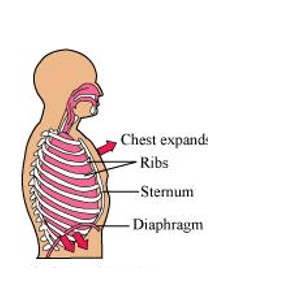Inspiration or inhalation is the process of bringing air from outside the body into the lungs. It is carried out by creating a pressure gradient between the lungs and the atmosphere.

When air enters the lungs, the diaphragm contracts toward the abdominal cavity, thereby increasing the space in the thoracic cavity for accommodating the inhaled air.

The volume of the thoracic chamber in the anteroposterior axis increases with the simultaneous contraction of the external intercostal muscles. This causes the ribs and the sternum to move out, thereby increasing the volume of the thoracic chamber in the dorsoventral axis.

The overall increase in the thoracic volume leads to a similar increase in the pulmonary volume. Now, as a result of this increase, the intra-pulmonary pressure becomes lesser than the atmospheric pressure. This causes the air from outside the body to move into the lungs.

Question 7: How is respiration regulated?

Solution. The respiratory rhythm centre present in the medulla region of the brain is primarily responsible for the regulation of respiration. The pneumotaxic centre can alter the function performed by the respiratory rhythm centre by signalling to reduce the inspiration rate.

The chemosensitive region present near the respiratory centre is sensitive to carbon dioxide and hydrogen ions. This region then signals to change the rate of expiration for eliminating the compounds.

The receptors present in the carotid artery and aorta detect the levels of carbon dioxide and hydrogen ions in blood. As the level of carbon dioxide increases, the respiratory centre sends nerve impulses for the necessary changes.

Question 8: What is the effect of $\mathrm{pCO}_{2}$ on oxygen transport?

Solution. $\mathrm{pCO}_{2}$ plays an important role in the transportation of oxygen. At the alveolus, the low $\mathrm{pCO}_{2}$ and high $\mathrm{pO}_{2}$ favours the formation of haemoglobin. At the tissues, the high $\mathrm{pCO}_{2}$ and low $\mathrm{pO}_{2}$ favours the dissociation of oxygen from oxyhaemoglobin. Hence, the affinity of haemoglobin for oxygen is enhanced by the decrease of $\mathrm{pCO}_{2}$ in blood. Therefore, oxygen is transported in blood as oxyhaemoglobin and oxygen dissociates from it at the tissues.

Question 9: What happens to the respiratory process in a man going up a hill?

Solution. As altitude increases, the oxygen level in the atmosphere decreases. Therefore, as a man goes uphill, he gets less oxygen with each breath. This causes the amount of oxygen in the blood to decline. The respiratory rate increases in response to the decrease in the oxygen content of blood. Simultaneously, the rate of heart beat increases to increase the supply of oxygen to blood

Question 10: What is the site of gaseous exchange in an insect?

Solution. In insects, gaseous exchange occurs through a network of tubes collectively known as the tracheal system. The small openings on the sides of an insect's body are known as spiracles. Oxygen-rich air enters through the spiracles. The spiracles are connected to the network of tubes. From the spiracles, oxygen enters the tracheae. From here, oxygen diffuses into the cells of the body.

The movement of carbon dioxide follows the reverse path. The $\mathrm{CO}_{2}$ from the cells of the body first enters the tracheae and then leaves the body through the spiracles.

Question 11: Define oxygen dissociation curve. Can you suggest any reason for its sigmoidal pattern?

Solution. The oxygen dissociation curve is a graph showing the percentage saturation of oxyhaemoglobin at various partial pressures of oxygen.

The curve shows the equilibrium of oxyhaemoglobin and haemoglobin at various partial pressures.

In the lungs, the partial pressure of oxygen is high. Hence, haemoglobin binds to oxygen and forms oxyhaemoglobin.

Tissues have a low oxygen concentration. Therefore, at the tissues, oxyhaemoglobin releases oxygen to form haemoglobin.

The sigmoid shape of the dissociation curve is because of the binding of oxygen to haemoglobin. As the first oxygen molecule binds to haemoglobin, it increases the affinity for the second molecule of oxygen to bind. Subsequently, haemoglobin attracts more oxygen.

Question 12: Have you heard about hypoxia? Try to gather information about it, and discuss with your friends.

Solution. Hypoxia is a condition characterised by an inadequate or decreased supply of oxygen to the lungs. It is caused by several extrinsic factors such as reduction in $\mathrm{pO}_{2}$, inadequate oxygen, etc. The different types of hypoxia are discussed below

Hypoxemic hypoxia

In this condition, there is a reduction in the oxygen content of blood as a result of the low partial pressure of oxygen in the arterial blood

Anaemic hypoxia

In this condition, there is a reduction in the concentration of haemoglobin.

Stagnant or ischemic hypoxia

In this condition, there is a deficiency in the oxygen content of blood because of poor blood circulation. It occurs when a person is exposed to cold temperature for a prolonged period of time.

Histotoxic hypoxia

In this condition, tissues are unable to use oxygen. This occurs during carbon monoxide or cyanide poisoning.

Question 13: Distinguish between

(a) IRV and ERV

(b) Inspiratory capacity and Expiratory capacity

(c) Vital capacity and Total lung capacity

Solution. (a)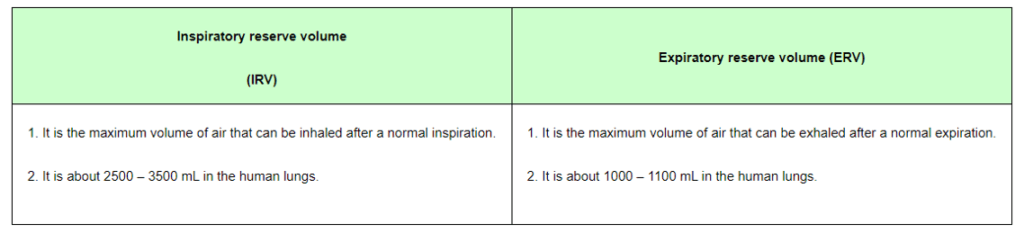(b)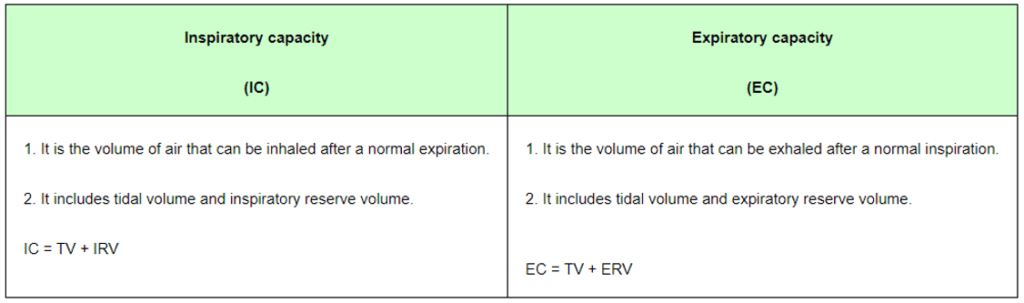(c)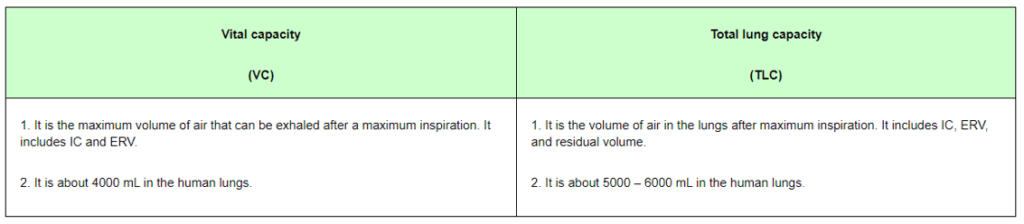Question 14: What is Tidal volume? Find out the Tidal volume (approximate value) for a healthy human in an hour. Solution. Tidal volume is the volume of air inspired or expired during normal respiration.

It is about 6000 to $8000 \mathrm{~mL}$ of air per minute.

The hourly tidal volume for a healthy human can be calculated as:

Tidal volume $=6000$ to $8000 \mathrm{~mL} /$ minute

Tidal volume in an hour $=6000$ to $8000 \mathrm{~mL} \times(60 \mathrm{~min})$

$=3.6 \times 10^{5} \mathrm{~mL}$ to $4.8 \times 10^{5} \mathrm{~mL}$

Therefore, the hourly tidal volume for a healthy human is approximately $3.6 \times 10^{5} \mathrm{~mL}$ to $4.8 \times 10^{5} \mathrm{~mL}$.